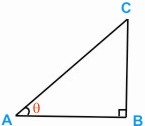HOME MATH DICTIONARY DOWNLOAD FEEDBACK DISCLAIMER
 Question: What is Sine of an Acute Angle ? Answer: The sine of an angle θ (sin θ as it is usually written) can be defined using a right-angled triangle, in which θ is one of the angles as shown, by sin θ = BC = Length of side opposite to θ AC length of hypotenuse.For example, if a ladder of length 5 metres leans against a wall at an angle of 65o to the horizontal, the height h metres at which it touches the wall is given by h/5 = sin 65o so h = 5 sin 65o = 5 x 0.906 307 8 = 4.531 538 9 (to calculator accuracy)